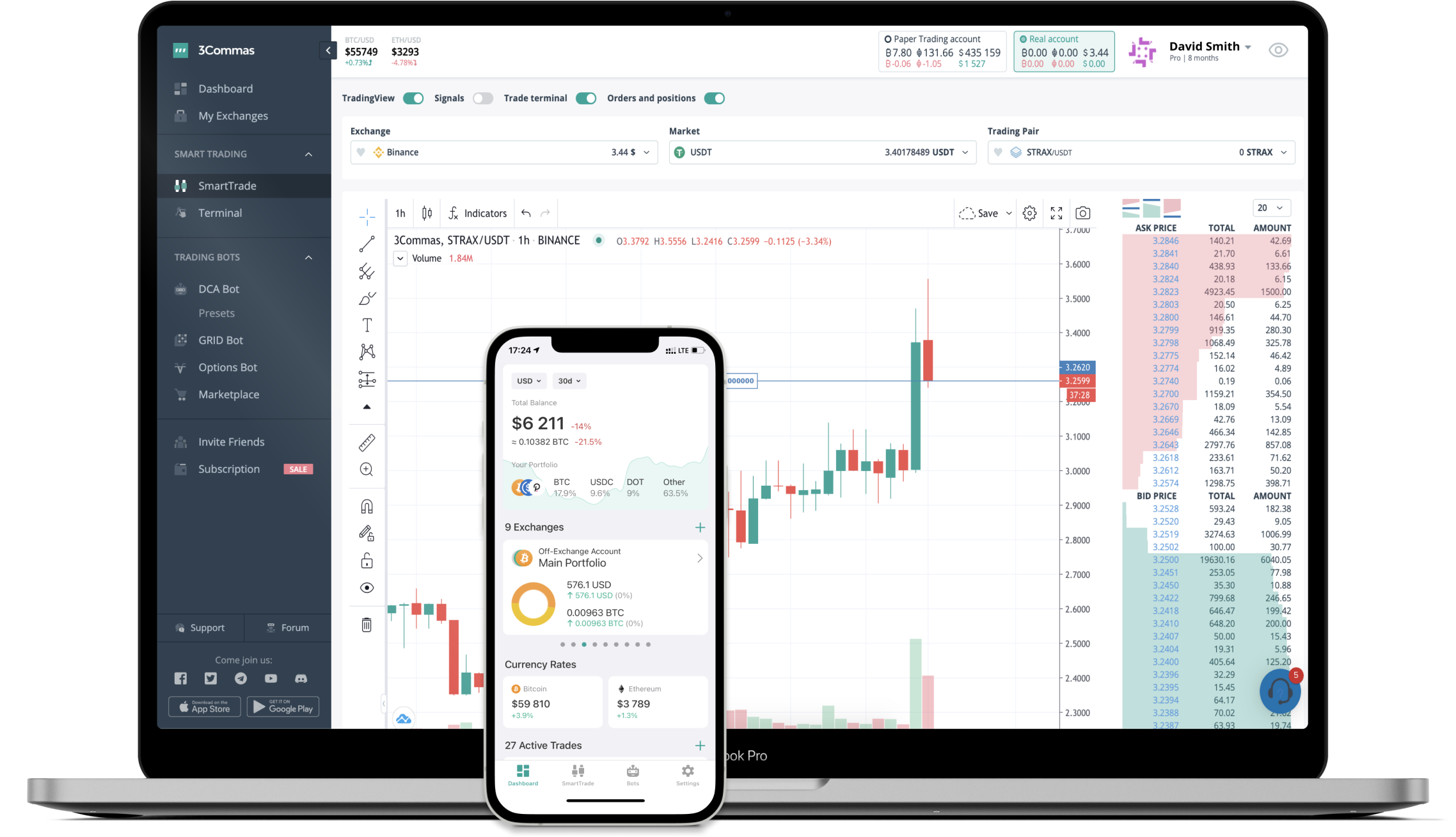Delta Financial
How it work

The 3Commas currency calculator allows you to convert a currency from Delta Financial (DELTA) to Canadian Dollar (CAD) in just a few clicks at live exchange rates.

Simply enter the amount of Delta Financial you wish to convert to CAD and the conversion amount automatically populates. You can also use our Prices Calculator Table to calculate how much your currency is worth in other denominations, i.e. .1 DELTA, .5 DELTA, 1 DELTA, 5 DELTA, or even 10 DELTA.

## DELTA to CAD Exchange Calculator

The chart displays Delta Financial price changes in CAD.

The ultimate tools for crypto traders to maximize trading profits while minimizing risk and loss.## Latest DELTA to CAD price calculator

Delta Financial market price is updated every three minutes and is automatically displayed in CAD. Below are the most popular denominations to convert to CAD.

Delta Financial
0.02
0.16
1.64
3.28
4.92
8.20
16.40
41.00
82.00
164.00
410.00
820.00
1640.00
4100.00
Delta Financial
0.00609756
DELTA
0.06097561
DELTA
0.60975610
DELTA
1.21951220
DELTA
1.82926829
DELTA
3.04878049
DELTA
6.09756098
DELTA
15.24390244
DELTA
30.48780488
DELTA
60.97560976
DELTA
152.43902439
DELTA
304.87804878
DELTA
609.75609756
DELTA
1524.39024390
DELTA

## FAQ

### How much is 1 Delta Financial in Canadian Dollar?

Delta Financial price in CAD is constantly changing.

At this moment, 1 Delta Financial equals 1.64 CAD

### How do I calculate the conversion from DELTA to CAD?

The 3Commas Delta Financial Calculator allows you to easily calculate the conversion price of DELTA to CAD by simply entering the amount of Delta Financial in the corresponding field and will automatically convert the value in Canadian Dollar (CAD).

You can also use our Delta Financial price table above to check the latest Delta Financial price in major fiat and crypto currencies.

### How can I convert Delta Financial to CAD?

The most common way of converting DELTA to CAD is by using a Crypto Exchange or a P2P (person-to-person) exchange platform like LocalBitcoins, etc.